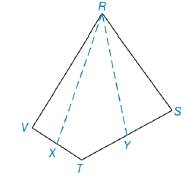Chapter 8.1, Problem 32E### Elementary Geometry for College St...

6th Edition
Daniel C. Alexander + 1 other
ISBN: 9781285195698

#### Solutions

Chapter
Section### Elementary Geometry for College St...

6th Edition
Daniel C. Alexander + 1 other
ISBN: 9781285195698
Textbook Problem
1 views

# For Exercise 31 and 32, X is the midpoint of V T ¯ and Y is the midpoint of T S ¯ . If A R Y T X = 13.5  in 2 , find A R S T V .To determine

To find:

ARSTV If ARYTX=13.5 in2.

Explanation

“A median of a triangle separated it into two triangles of equal area”.

Calculation:

Given,

Area of RYTX(A)=13.5 in2

Draw a diagonal RT¯ in RSTV which separates RSTV into two triangle RVT and RVT

In RVT X is the midpoint of VT¯ from R

(i.e.) RX is the median of RVT since, “A median of a triangle separated it into two triangles of equal area”

(i.e.)RX¯ separates the RVT into RXV and RXT with equal area,

ARXV=ARXT

In RTS,Y is the midpoint of TS¯ from R.

(i.e.) RY¯ is the median of RTS

RY¯ separates the RTS into RYT and RYS with equal area,

ARYT=ARYS

Now, the figure becomes,

### Still sussing out bartleby?

Check out a sample textbook solution.

See a sample solution

#### The Solution to Your Study Problems

Bartleby provides explanations to thousands of textbook problems written by our experts, many with advanced degrees!

Get Started

#### What are the two requirements for a random sample?

Statistics for The Behavioral Sciences (MindTap Course List)

#### Sketch the graphs of the equations in Exercises 512. yx=1

Finite Mathematics and Applied Calculus (MindTap Course List)

#### In Problems 29-36, find the particular solution to each differential equation.

Mathematical Applications for the Management, Life, and Social Sciences

#### Suppose . Then F(x)g(x) + C f′(g(x)) + C F(g′(x)) + C F(g(x)) + C

Study Guide for Stewart's Single Variable Calculus: Early Transcendentals, 8th

#### Identify and describe the steps of the scientific method.

Research Methods for the Behavioral Sciences (MindTap Course List)